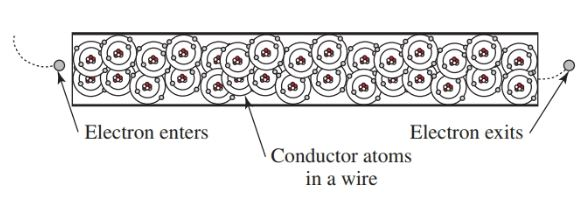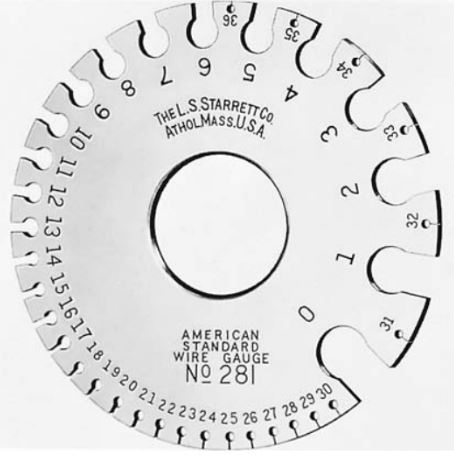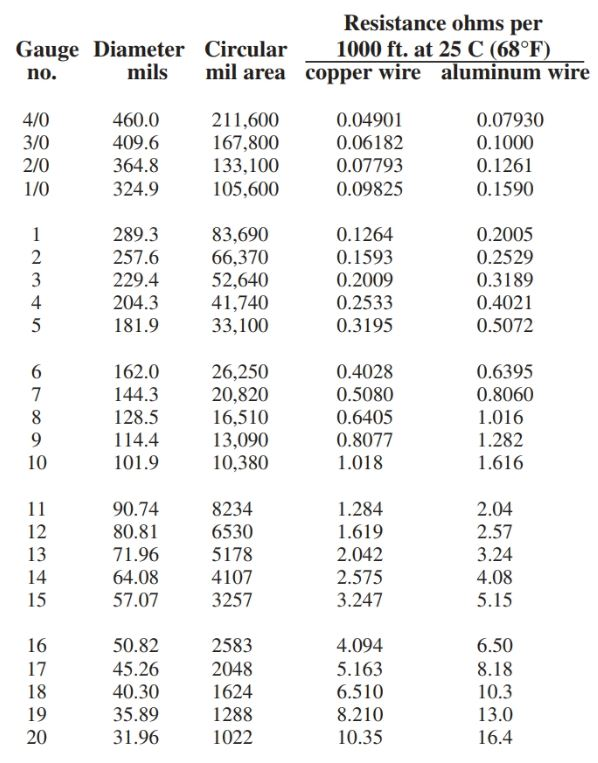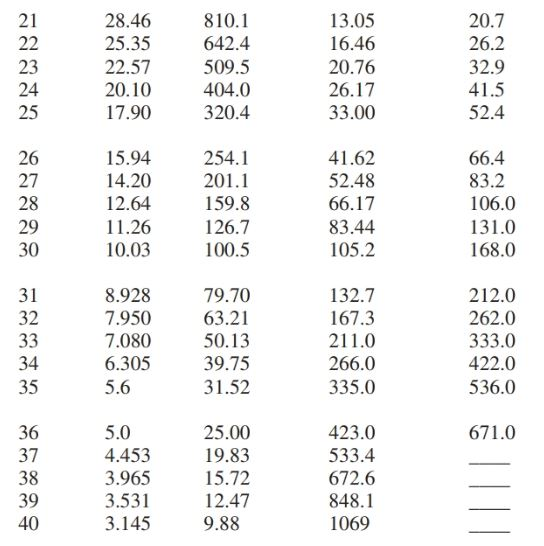Home / Electrical Circuits / Wire Gauge Sizes | Chart | Circular Mil Area

Wire Gauge Sizes | Chart | Circular Mil Area

Want create site? Find Free WordPress Themes and plugins.

Conductors are the pathways that allow electrons to flow through an electrical circuit. Conductors can be made in various shapes and sizes. They can be made from a number of different materials.

The most common conductor you will encounter is copper wiring. Copper is an excellent conductor and can be formed into many different shapes.

Copper comes in round, square, stranded, solid, flat ribbon, and bar shapes. Each shape has its own unique qualities that make it best for use in some specific application.

Conductors vary depending on the type of material from which they are made. Copper is an excellent conductor.

Silver is an even better conductor. However, silver is too expensive to be used in common circuits.

Many high-voltage lines are made from aluminum because of its lightweight, and they have a center core made of stranded steel for added strength.

Brass is used for electrical-mechanical equipment such as switch parts because of its strength and conductive properties.

The actual conduction of electricity is done by transferring electrons from one atom to the next atom in the conductor.

Assume that a piece of copper wire is neutral. If an electron is forced into one end of the wire, then an electron will also be forced out from the other end, Figure 1.Figure 1. When an electron enters one end of a conductor, another electron is forced out the other end. This all happens at approximately the speed of light.

The original electron did not flow through the conductor. Yet the energy was transferred by the interaction between the electrons in the conductor.

The actual transfer of electrical energy occurs at an amazing speed. The speed has been accurately measured, and it approaches the speed of light: 186,000 miles per second! In metric terms, this speed is 300,000 kilometers (or 300,000,000 meters) per second.

The unit for measuring conductance is the Siemens. This unit was named after the German inventor Ernst von Siemens, who did a great deal of work in the development of telegraphy use.

The abbreviation for the Siemens is the capital letter S. The unit formula for computing electrical conductance is as follows:

$G=\frac{1}{R}$

Where G is conductance (in Siemens) and R is resistance (in ohms).

The conductance of a material is the reciprocal of the resistance of the material.

Conductor Sizes

Conductors are sized by their cross-sectional area. The amount of cross-sectional area a conductor has determined how much current it can handle without overheating.

American Wire Gauge System

Circular conductors are arranged according to size by the American Wire Gauge System. The larger the gauge number, the smaller the cross-sectional area a wire will have. For example, a No. 14 wire is larger in diameter than a No. 20 wire. See Figure 2.Figure 2. A gauge is used to find wire size.

This system of wire sizes was developed over a hundred years ago. At that time, no one could foresee that the demand for electrical products would make this system obsolete.

Some wire sizes are now larger than the No. 1 conductor. A temporary solution to this problem created the next set of larger sizes. The next four sizes that were developed are 0 (pronounced “ought”), 00, 000, and 0000–or one through four ought (1/0 through 4/0).

Circular Mil Area

When it became apparent that even larger sizes would be needed, the final solution to the sizing problem, the third set of sizes, was developed.

The final system of sizing is the circular mil system. The circular mil system is based on the diameter of the conductor measured in mil (1/1000 of an inch). Typical conductors larger than four ought are from 250 kcm to 2000 kcm.

The letters kcm represent 1000 circular mil, so a 250 kcm represents 250,000 circular mil. Figure 3 contains all the wire sizes you would normally encounter.Figure 3. Wire Gauge Sizes Chart

Circular mil area is the common way to express the cross-sectional area of a conductor. As previously noted, one mil is equal to 1/1000th of an inch (0.001 inches).

The number of the circular mil (cmil) in a conductor is equal to the diameter of a round conductor squared (D2), where D is in mil. Therefore, a wire with the diameter of 1 mil has an area of 1 circular mil (1 cmil). A wire with a diameter of 2 mil has an area of 4 cmil (2 × 2 = 4). A wire with a diameter of 15 mils gives us an area of 225 cmil (15 × 15 = 225). See Figure 4.Figure 4. The area in circular mil is found by squaring the diameter in mil.

The circular mil is a more convenient method of expressing the size of a conductor than πr2, which is used to compute the area of a circle.

If we must find the area of a square or rectangular conductor, their equivalent in cmil is not difficult to find. To find the circular mil equivalent, compute the square/rectangular conductor’s area in square mil and then divide that area by 0.7854.

For example, if a 1″ by 1/4″ bar of copper is to be used as a conductor:

• First, convert the inch measurements to mil (1″ = 1000 mil and 1/4″ = 250 mils).
• Then multiply the dimensions in mil to get the area in square mil (1000 × 250 = 250,000 sq. mil).
• Finally, divide the square mil area by 0.7854 (250,000/0.7854 = 318,309).
• This results in the original 1″ by 1/4″ copper bar having a surface area equivalent to a round conductor of 318,309 cmil.

Wire charts commonly express the electrical values for conductors solely based on their size in cmil. To determine the current carrying capacity, or resistance value, of a rectangular conductor, the rectangular conductor must be converted to circular mil.

Conductor Resistance

Even though conductors provide a low-resistance path for electron flow, they still have some resistance. This resistance must be considered when long distances are involved. There are four factors directly relating to the resistance of conductors.

• A cross-sectional area of the conductor. The larger the surface area or diameter of a conductor, the lower the resistance.
• Type of conductor material. Different materials have different resistance values.
• Length of the conductor. The longer the conductor, the greater the resistance.
• The temperature of the material. The resistance of a material rises with rising temperature.

Cross-Sectional Area of a Conductor

Increasing the cross-sectional area of a conductor increases the amount of current that can flow. To help visualize this, use the flow of water through a pipe as an example, Figure 5.

A large-diameter pipe can carry more gallons of water per minute than a small-diameter pipe. An electric conductor operates in the same fashion. The large-diameter conductor carries more electrons per minute than a small-diameter conductor.Figure 5. The larger the diameter of a pipe, the more gallons flow per minute. The larger the diameter of a conductor, the greater the current and the lower the resistance.

Type of Material

The type of material affects conductance and resistance. Figure 6 shows one example. A No. 12 copper wire has 1.619 ohms resistance per 1000 feet. An aluminum conductor of the same diameter and length offers 2.57 ohms of resistance.Figure 6. Two conductors of equal diameter will have different resistance values when made from different types of material. No. 12 copper wire has less resistance than a No. 12 aluminum wire.

Length of Conductor

The length of a conductor greatly affects its total resistance. If one foot of wire has a certain resistance, then ten feet of the same wire will have ten times more resistance. Fifty feet of the wire will have fifty times more resistance, and so on.

As a conductor becomes longer, it creates a voltage drop in a circuit. A circuit using short lengths of wire, such as an electrical lab project using three to six inches of No. 22 wire, does not create a major problem. But long runs of wiring can create electrical problems.

In Figure 7, a 10-amp load is connected to a circuit using No. 22 copper wire. The load is at a distance of 100 feet from the source. When the switch to the motor is closed to connect the motor, the motor will heat up internally because an insufficient voltage is being applied.

The low voltage is a result of the voltage drop along the length of the conductor. The loss of voltage can be computed using Ohm’s law.Figure 7. The voltage drop caused by the resistance of 200 feet of No. 22 copper conductor connected to a 10 amp load is equal to 32.9 volts, or 16.45 volts for every 100 feet of conductor.

Wire Size and Voltage Drop

The electrons must travel a total distance of 200 feet to make a complete circuit. The total resistance of the wiring is equal to 3.29 Ω. The current through the load is 10 amps. By applying Ohm’s law to these conditions, a voltage drop equal to 32.9 volts has been created (E = 10 A × 3.29 Ω). Subtracting the 32.9-volt drop from the source voltage of 120 leaves only 87.1 volts for the load. This amount of voltage is undesirable, and will no doubt cause equipment failure, especially for an electrical motor load, such as a drill.

Did you find apk for android? You can find new Free Android Games and apps.